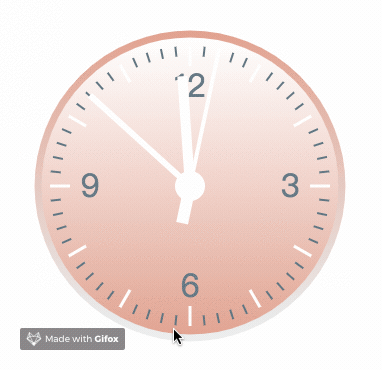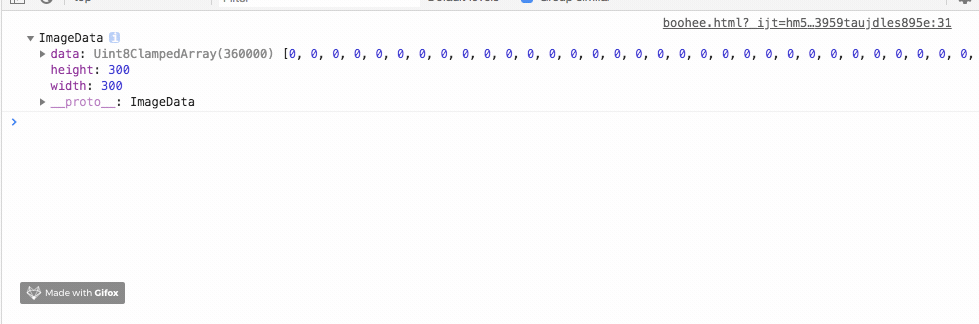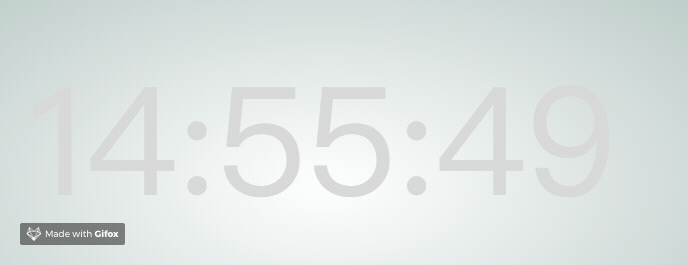# canvas中普通动效与粒子动效的实现代码示例

发布时间：2019-01-03 15:19:47   作者：薄荷前端我要评论

canvas用于在网页上绘制图像、动画，可以将其理解为画布，在这个画布上构建想要的效果。本文详细的介绍了粒子特效，和普通动效进行对比，非常具有实用价值，需要的朋友可以参考下

canvas用于在网页上绘制图像、动画，可以将其理解为画布，在这个画布上构建想要的效果。

canvas可以绘制动态效果，除了常用的规则动画之外，还可以采用粒子的概念来实现较复杂的动效，本文分别采用普通动效与粒子特效实现了一个简单的时钟。.save()表示保存canvas当前环境的状态，在此基础上进行绘制。绘制完成之后，返回之前保存过的路径状态和属性。

```  // 小时时间刻度
offscreenCanvasCtx.save();
for (var i = 0; i < 12; i++) {
offscreenCanvasCtx.beginPath();
// 刻度颜色
offscreenCanvasCtx.strokeStyle = '#fff';
// 刻度宽度
offscreenCanvasCtx.lineWidth = 3;
// 每小时占据30°
offscreenCanvasCtx.rotate(Math.PI / 6);
// 开始绘制的位置
offscreenCanvasCtx.lineTo(140, 0)
// 结束绘制的位置;
offscreenCanvasCtx.lineTo(120, 0);
// 绘制路径
offscreenCanvasCtx.stroke();
}
offscreenCanvasCtx.restore();```

```  var now = new Date(),
sec = now.getSeconds(),
min = now.getMinutes(),
hr = now.getHours();
hr = hr > 12 ? hr - 12 : hr;

//秒针
offscreenCanvasCtx.save();
offscreenCanvasCtx.rotate(sec * (Math.PI / 30));
......
offscreenCanvasCtx.stroke();```

canvas可以用来绘制复杂，不规则的动画。粒子特效可以用来实现复杂、随机的动态效果。```let image = new Image();
image.src='../image/logo.png';
let pixels=[]; //存储像素数据
let imageData;
image.width = 300;
image.height = 300
// 渲染图片，并获取该区域内像素信息
ctx.drawImage(image,(canvas.width-image.width)/2,(canvas.height-image.height)/2,image.width,image.height);
imageData=ctx.getImageData((canvas.width-image.width)/2,(canvas.height-image.height)/2,image.width,image.height); //获取图表像素信息
//绘制图像
};`````` function getPixels(){
var pos=0;
var data=imageData.data; //RGBA的一维数组数据
//源图像的高度和宽度为300px
for(var i=1;i<=image.width;i++){
for(var j=1;j<=image.height;j++){
pos=[(i-1)*image.width+(j-1)]*4; //取得像素位置
if(data[pos]>=0){
var pixel={
x:(canvas.width-image.width)/2+j+Math.random()*20, //重新设置每个像素的位置信息
y:(canvas.height-image.height)/2+i+Math.random()*20, //重新设置每个像素的位置信息
fillStyle:'rgba('+data[pos]+','+(data[pos+1])+','+(data[pos+2])+','+(data[pos+3])+')'
}
pixels.push(pixel);
}
}
}
}
function drawPixels() {
var canvas = document.getElementById("myCanvas");
var ctx = canvas.getContext("2d");
ctx.clearRect(0,0,canvas.width,canvas.height);
var len = pixels.length, curr_pixel = null;
for (var i = 0; i < len; i++) {
curr_pixel = pixels[i];
ctx.fillStyle = curr_pixel.fillStyle;
ctx.fillRect(curr_pixel.x, curr_pixel.y, 1, 1);
}
}```

``` function time() {
ctx.clearRect(0,0,canvas.width,canvas.height)
ctx.font = "150px 黑体";
ctx.textBaseline='top';
ctx.fillStyle = "rgba(245,245,245,0.2)";
ctx.fillText(new Date().format('hh:mm:ss'),(canvas.width-textWidth)/2,(canvas.height-textHeight)/2,textWidth,textHeight);
}``````  function getPixels(){
let imgData = ctx.getImageData((canvas.width-textWidth)/2,(canvas.height-textHeight)/2,textWidth,textHeight);
let data = imgData.data
pixelsArr = []
for(let i=1;i<=textHeight;i++){
for(let j=1;j<=textWidth;j++){
pos=[(i-1)*textWidth+(j-1)]*4; //取得像素位置
if(data[pos]>=0){
var pixel={
x:j+Math.random()*20, //重新设置每个像素的位置信息
y:i+Math.random()*20, //重新设置每个像素的位置信息
fillStyle:'rgba('+data[pos]+','+(data[pos+1])+','+(data[pos+2])+','+(data[pos+3])+')'
};
pixelsArr.push(pixel);
}
}
}
}```

`imgData`保存了所选区域内的像素信息，每个像素点占据4位，保存了RGBA四位信息。筛选每个像素的第四位，这段代码中将所有透明度不为0的像素都保存到了数组`pixelsArr`中。

`x``y`记载了该粒子的位置信息，为了产生效果图中的运动效果，给每个粒子添加了0-20个像素的偏移位置，每次重绘时，偏移位置随机生成，产生运动效果。

```  function drawPixels() {
// 清除画布内容，进行重绘
ctx.clearRect(0,0,canvas.width,canvas.height);
for (let i in pixelsArr) {
ctx.fillStyle = pixelsArr[i].fillStyle;
let r = Math.random()*4
ctx.fillRect(pixelsArr[i].x, pixelsArr[i].y, r, r);
}
}```

```  function time() {
......
getpixels(); //获取粒子
drawPixels(); // 重绘粒子
requestAnimationFrame(time);
}```

`window.requestAnimationFrame(callback)` 方法告诉浏览器您希望执行动画并请求浏览器在下一次重绘之前调用指定的函数来更新动画。该方法使用一个回调函数作为参数，这个回调函数会在浏览器重绘之前调用。